# Mechanical Engineering - Engineering Mechanics

### Exercise :: Engineering Mechanics - Section 1

21.

The force required to move the body up the plane will be minimum if it makes an angle with the inclined plane __________ the angle of friction.

 A. equal to B. less than C. greater than

Explanation:

No answer description available for this question. Let us discuss.

22.

In the shown figure, if the angle of inclination of the plane is increased, then acceleration of the system will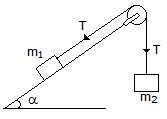A. increase B. decrease C. remain the same

Explanation:

No answer description available for this question. Let us discuss.

23.

The total time taken by a projectile to reach maximum height and to return back to the ground, is known as time of flight.

 A. Yes B. No

Explanation:

No answer description available for this question. Let us discuss.

24.

The frequency of oscillation of a torsional pendulum is

 A.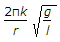B.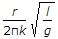C.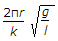D.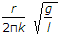Explanation:

No answer description available for this question. Let us discuss.

25.

The range of a projectile is maximum, when the angle of projection is

 A. 30° B. 45° C. 60° D. 90°